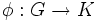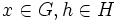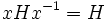# Normal subgroup

The article on this topic in the Group Properties Wiki can be found at: normal subgroupTemplate:Subgroup property

## Definition

A subgroup$H$ of a group$G$ is termed normal if it satisfies the following equivalent conditions:

•$H$ is the kernel of a homomorphism from$G$, i.e. there is a homomorphism$\phi:G \to K$ of groups such that$\phi^{-1}(e) = H$
•$xHx^{-1} \subseteq H$, or in other words,$xhx^{-1} \in H$ for all$x \in G, h \in H$
•$xHx^{-1} = H$